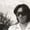FREELessons: 9Length: 37 minutes• Overview
• Transcript

# 2.4 Map, Filter, and Reduce Operations

In this lesson, you'll learn how to create longer streams of chains to perform more advanced operations. While doing so, you'll learn how to adopt the functional programming paradigm in your Android apps.

## 3.Conclusion1 lesson, 00:39

### 2.4 Map, Filter, and Reduce Operations

Welcome back, in the previous lesson, I introduced you to streams, and we performed a few basic operations using them. But all of those operations could have been easily done with loops and arrays or lists statically. You really didn't get to know the real flexibility and ease that the stream API can offer. In this lesson, I'll try to show you just that. Let us now try to create a longer chain of stream operations. Let's say we want to first calculate the square root of all the elements in the array. And then print only those square roots that are greater than the number 4. So you gotta start by creating a new stream for the array. We can't use this number stream because we have used it already. You have to always remember that a stream can be used only once. If you try using it multiple times, you will get a runtime error. Next, you might have guessed that we need to use the math method to calculate the square root of the elements. So you would have to write code that looks like this. But this is not allowed because the square root method returns a double. You could manually convert the double to int before returning it, but there's another approach. You could convert the entire integer stream into a double stream using the asDoubleStream method. And now you can call the math method as usual. Next, we want to create a new screen that contains only those square roots that are greater than 4. For this kind of operation, which involves moving certain elements from a screen, you must use the figure method, which is quite aptly named. The lambda you pass to this method should return a boolean value based on its imput. If the return value is true, the input is retained. But if the return value is false, the input is discarded. In our case, we want to retain only those square roots that are greater than 4, so you can add an appropriate condition here. At this point, we have a stream containing only the square root we wanted, so we can print it. If you run the app now, you should be able to see the results. For the sake of another example, let's try to filter the array of strings so that it contains only those strings that start with W. So start by creating a new stream for it, then call the filter method. And in the expression lambda, you just have to use the startsWith method. This new stream will now have only two strings. Let me quickly add code to log them. And now, the last string method we will be looking at is called the reduce method. All this while, we have been using methods that worked independently on all the individual elements of the stream. Reduce is a method that allows you to generate a single result by combining all the elements of the stream. For example, let's say you want to concatenate all the strings in our array of strings. So first, you create a new stream for it, and then you call the reduce method. As its input, this method expects a starting value and a lambda. The starting value depends on the operation that you are performing inside the lambda. You're trying to perform a concatenation operation. So the starting value can be an empty string. Our lambda will always accept two arguments. Both of them in this case will be strings. The first argument is a partial result of the reduction operation, and the second argument is the next element of the stream. So the idea here is that the next element will be concatenated to the partial result. So we can simply say x.concat(y) here. At this point, we no longer have a stream. We'll actually have a single result which for now is a string. Let's log it to see what it contains. As expected, we have a large string made up of the smaller strings in the stream. To give you another example, let's try to find the product of all the numbers in our numbers array. So we create a new stream for it, and then we call the reduce method again. This time, the starting value must be 1, because 1 is the identity element for multiplication. By the way, if you were trying to find the sum of all the elements, you would have used 0 as the identity element. All right, now in the lambda, all you need to do is return x multiplied with y. Let's try printing that as well. There you go, the product looks correct. You now know how to use streams and perform a variety of operations on them. You'll need a bit of practice to get comfortable with them. Because using them involves a functional programming approach. But once you do master them, I'm sure you won't want to go back to using loops, thanks for watching.

Back to the top